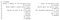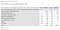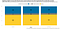# Applying Weights

## What difference does applying survey weights make?

Weights adjust survey responses, when producing survey statistics.

This article looks at R code for applying survey weights. We find the difference that applying those weights make.

# Importing the data

This article uses European Social Survey data for the United Kingdom. NatCen conducted the ninth wave, interviewing 2,204 UK adults aged 15 or over. These face-to-face interviews were between 31st August 2018 and 22nd February 2019.

If there were to be a new referendum tomorrow, would you vote for the UK to remain a member of the European Union or leave the European Union?

First, let’s import the SAS data file into R, with the haven package:

`ESS09GB_sav <- read_sav("ESS9GB.sav")`

In its current form, responses to the EU referendum vote intention question look like:

• 1: Remain a member of the European Union;
• 2: Leave the European Union;
• 33: Would submit a blank ballot paper.

There are other coded responses too. The data file does not code unsure or refused responses. This is not as useful as it could be. We need to replace the enumerated responses with what they mean in the code book:

`ESS09GB_sav_df <- ESS09GB_sav %>%   mutate_all(sjlabelled::as_label)`

This line replaces values with their labels, which is good for our question.

# Survey design and tables

One means of calculating survey statistics is using Thomas Lumley’s survey package. In that package, we need to specify the survey design.

One example of survey weights is for respondent sex. Suppose we had 600 responses from men, and 400 from women. Women are not 40% of the population. We need to count the responses from women more, and men less. The aim is for the weighted response is to reflect the population. The sample should look like a small but scaled version of our population.

There are three designs we could use:

• Unweighted: This is the basic counts of each response. By definition, every person counts for exactly one unit.
• Design: design weights adjust for different probabilities of selection.
• Post-stratification: these weights adjust for selection probabilities, some sampling error and non-response bias.

In general, we would not use unweighted statistics.

`ess09gb_unweighted <- svydesign(data = ESS09GB_sav_df,                                ids = ~1, weights = NULL)ess09gb_poststrat <- svydesign(data = ESS09GB_sav_df,                               ids = ~1, weights = ~pspwght)`

The survey table function then produces the required statistics:

`svytable(formula = ~vteumbgb,         design = ess09gb_unweighted, Ntotal = 100)svytable(formula = ~vteumbgb,         design = ess09gb_poststrat, Ntotal = 100)`

Under post-stratification weights, the Remain share estimate was 57%:This is the survey table. (Image: R Pubs)

The table mirrors outputs from the ESS portal, suggesting we did this right.The Remain estimate is, again, 57%. (Image: ESS Data Portal)

# Direct calculation

Another means is direct calculation the figures. Some users may prefer this method, if we need to compare different weights.

As you would expect, the code is more involved:

`ESS09GB_EUmem_df <- ESS09GB_sav_df %>%  filter(cntry == "United Kingdom" &vteumbgb != "NA") %>%  group_by(vteumbgb) %>%  summarise(unweighted = n(),            design = sum(dweight),            post_strat = sum(pspwght)) %>%  mutate(unweighted = 100*unweighted/sum(unweighted),         design = 100*design/sum(design),         post_strat = 100*post_strat/sum(post_strat))`

We can group the non-affirmative responses together:

`ESS09GB_EUmem_tidy_df <- ESS09GB_EUmem_df %>%  mutate(EUmembershipchoice =           case_when(vteumbgb == "Remain a member of the European Union" ~ "Remain",                     vteumbgb == "Leave the European Union" ~ "Leave",                     TRUE ~ "Would Not Valid Vote")) %>%  group_by(EUmembershipchoice) %>%  summarise(Unweighted = sum(unweighted),            Design = sum(design),            "Post-Stratification" = sum(post_strat)) %>%  pivot_longer(cols = 2:4,               names_to = "Weights",               values_to = "Share")`

Finally, we can put together a graph. It shows the increased Remain share under the applied weights.One downside is that this graph does not show the uncertainty. (Image: R Pubs)

Survey provides estimates, subject to many sources of potential error. Processing error includes inappropriate survey weights.

The R code is available on GitHub and R Pubs. The GitHub folder includes the SAS data file.

This blog looks at the use of statistics in Britain and beyond. It is written by RSS Statistical Ambassador and Chartered Statistician @anthonybmasters.

## More from Anthony B. Masters

This blog looks at the use of statistics in Britain and beyond. It is written by RSS Statistical Ambassador and Chartered Statistician @anthonybmasters.